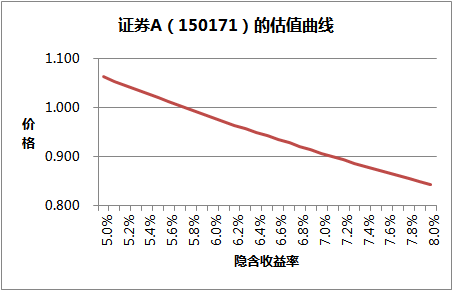# 分级基金 A 级的估值模型

### 1. 永续债的隐含收益率

$$p=\sum_{i=0}^{+\infty}\frac{c}{(1+r)^{i+t}}=\frac{c(1+r)^{1-t}}r$$

$$p\approx\frac{cn}{r}$$

$$r\approx\frac{cn}{p}$$

### 2. 考虑票息浮动的隐含收益率

$$\begin{array}{rcl}p&=&\frac{c_0}{(1+r)^t}+\sum_{i=1}^{+\infty}\frac{c_1}{(1+r)^{i+t}}\\ &=&\frac{c_1(1+r)^{1-t}}{r}+\frac{c_0-c_1}{(1+r)^t}\\ &\approx&\frac{c_1n}{r}+{c_0-c_1} \end{array}$$

$$r\approx\frac{c_1n}{p-(c_0-c_1)}$$

### 3. 再考虑一些细节

$$\begin{array}{rcl}p&=&\frac{n-1+c_0t}{(1+r)^t}+\sum_{i=1}^{+\infty}\frac{c_1}{(1+r)^{i+t}}\\ &=&\frac{c_1(1+r)^{1-t}}{r}+\frac{n-1+c_0t-c_1}{(1+r)^t}\end{array}$$

### 4. 考虑下折和上折后的隐含收益率

• 母基金的波动性越大， A 类价值越高（同样价格下，隐含收益率越高），因为触发下折的概率越大。
• 下折触发点越小， A 类价值越低，因为触发下折的难度变大。
• 若 B 级单位净值越接近下折触发点， A 级的价值越高。
• 申万收益（ 150022 ）无低折，所以没有低折带来的期望收益。所以它的折价率一直比较高。

### 5. 以证券 A （ 150171 ）为例Q. E. D.

##### 系列： 低风险投资 »

12 月上旬，分级基金走出了波澜壮阔的大行情，部分基金的规模上升 100+倍，也让分级基金进入越来越多投资者的视野。这篇文章简单介绍什么是分级基金、分级基金的定价以及最近很火的套利交易。

##### 类似文章：
12 月上旬，分级基金走出了波澜壮阔的大行情，部分基金的规模上升 100+倍，也让分级基金进入越来越多投资者的视野。这篇文章简单介绍什么是分级基金、分级基金的定价以及最近很火的套利交易。

12 月上旬，分级基金走出了波澜壮阔的大行情，部分基金的规模上升 100+倍，也让分级基金进入越来越多投资者的视野。这篇文章简单介绍什么是分级基金、分级基金的定价以及最近很火的套利交易。